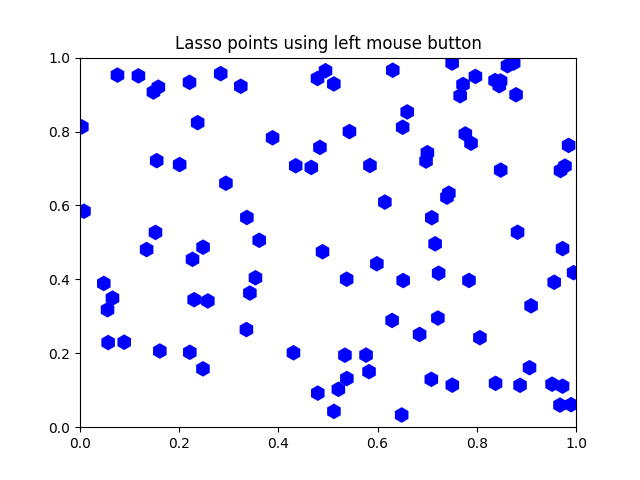# Lasso Demo¶

Show how to use a lasso to select a set of points and get the indices of the selected points. A callback is used to change the color of the selected points

This is currently a proof-of-concept implementation (though it is usable as is). There will be some refinement of the API.```from matplotlib import colors as mcolors, path
from matplotlib.collections import RegularPolyCollection
import matplotlib.pyplot as plt
from matplotlib.widgets import Lasso
import numpy as np

class Datum(object):
colorin = mcolors.to_rgba("red")
colorout = mcolors.to_rgba("blue")

def __init__(self, x, y, include=False):
self.x = x
self.y = y
if include:
self.color = self.colorin
else:
self.color = self.colorout

class LassoManager(object):
def __init__(self, ax, data):
self.axes = ax
self.canvas = ax.figure.canvas
self.data = data

self.Nxy = len(data)

facecolors = [d.color for d in data]
self.xys = [(d.x, d.y) for d in data]
self.collection = RegularPolyCollection(
6, sizes=(100,),
facecolors=facecolors,
offsets=self.xys,
transOffset=ax.transData)

self.cid = self.canvas.mpl_connect('button_press_event', self.onpress)

def callback(self, verts):
facecolors = self.collection.get_facecolors()
p = path.Path(verts)
ind = p.contains_points(self.xys)
for i in range(len(self.xys)):
if ind[i]:
facecolors[i] = Datum.colorin
else:
facecolors[i] = Datum.colorout

self.canvas.draw_idle()
self.canvas.widgetlock.release(self.lasso)
del self.lasso

def onpress(self, event):
if self.canvas.widgetlock.locked():
return
if event.inaxes is None:
return
self.lasso = Lasso(event.inaxes,
(event.xdata, event.ydata),
self.callback)
# acquire a lock on the widget drawing
self.canvas.widgetlock(self.lasso)

if __name__ == '__main__':

np.random.seed(19680801)

data = [Datum(*xy) for xy in np.random.rand(100, 2)]
ax = plt.axes(xlim=(0, 1), ylim=(0, 1), autoscale_on=False)
ax.set_title('Lasso points using left mouse button')

lman = LassoManager(ax, data)

plt.show()
```

Gallery generated by Sphinx-Gallery